Next: MINIMUM ROUTING TREE CONGESTION Up: Spanning Trees Previous: MINIMUM GEOMETRIC STEINER TREE   Index

### MINIMUM GENERALIZED STEINER NETWORK

• INSTANCE: Graph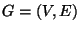, a weight function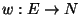, a capacity function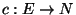, and a requirement function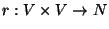.

• SOLUTION: A Steiner network over G that satisfies all the requirements and obeys all the capacities, i.e., a function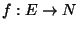such that, for each edge e,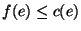and, for any pair of nodes i and j, the number of edge disjoint paths between i and j is at least r(i,j) where, for each edge e, f(e) copies of e are available.

• MEASURE: The cost of the network, i.e.,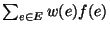.

• Good News: Approximable within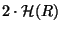where R is the maximum requirement and, for any n,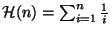.

• Comment: Also called Minimum Survivable Network. Approximable within 2 when all the requirements are equal . If the requirements are equal and the graph is directed the problem is approximable within. The variation in which there are no capacity constraints on the edges is approximable within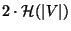. This problem belongs to the class of problems of finding a minimum-cost subgraph such that the number of edges crossing each cut is at least a specified requirement, which is a function f of the cut. If f is symmetric and maximal then any problem in this class is approximable within 2R, where R is the maximum value of f over all cuts .Next: MINIMUM ROUTING TREE CONGESTION Up: Spanning Trees Previous: MINIMUM GEOMETRIC STEINER TREE   Index
Viggo Kann
2000-03-20